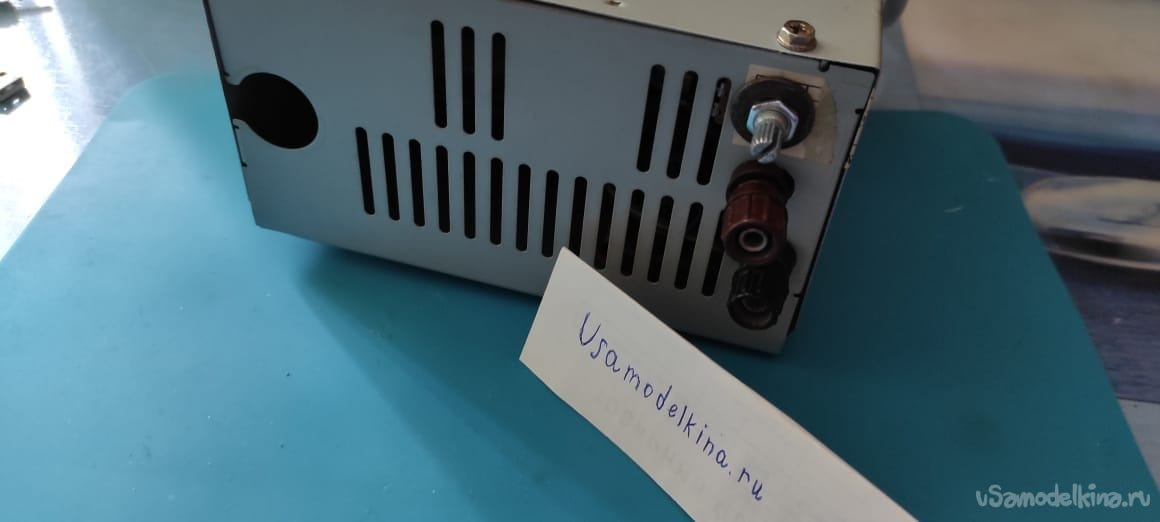DIY

# TL431 laboratory power supplyHello everyone, today we will make a laboratory power supply in the case of an old ATX computer power supply. He's not perfect but has the right to live!
The technical characteristics of the device are as follows: Stabilized adjustable output voltage 2.7-17V Adjustable output current 0-3A (5A) Power 50 (80) W Linear diagram
Tools and materials:
– Defective ATX power supply
– Capacitors 2pcs 100nF
-Capacitors 1pc 10μF
-Capacitors 2pcs 2200mkF
-Powerful transistor npn 3pcs MJE13007
-Transistor KT817 (kt815) BD139
-Resistors of different ratings and powers
-12V fan
-12V Zener diode
-Diodes 1N4007
Voltammeter
Chip TL431
-Transistor 1pc 2N5551
-Variable resistor 1pc 1K
-Variable resistor 1pc 10K
-Fuse 2A
-Transformer TN-7 (any TN) or other
-Diode bridge at least 6A
-Soldering iron
-Solder
-Flux
Scheme:
Diodes D1 and D2 are 1N4007, C6 and C7 at 100nF. T1 and T5, T3 any powerful n-p-n transistor (13007).
R6 is a 0.22 Ohm shunt, T2 is KT817 (KT815, BD139). And T4 is 2N5551, Br1 is a diode bridge for 6A or more!
P1 for 10K, P2 for 1K.R2 for 240Ω 2W, R4 for 1KΩ 1W and R8 10KΩ 2W (5W).D4 is a 12V zener diode, a 12V cooler. R7 for 220 Ohm (not 1KOM), also Tr1 transformer TN-7 or any other!
Creation
Let's disassemble the ATX power supply and take the case and radiator, a cooler, possibly also a diode bridge.
We put a diode bridge and a transistor on thermal paste!We solder the capacitor and the heatsink with the details on the breadboard. We drill holes for glue, transformer and potentiometer.Bolt the transformer into the case, solder the primary winding through the fuse to the cable.
Before that, connect the windings for 220V and 12.6V.We solder the circuit and the cooler.We fasten the terminals and the potentiometer, close the lid! We connect to the network.Formulas and Calculations
First you need to determine the resistance of the resistor. With a maximum input voltage of 19V according to Ohm's law, the resistance is calculated as follows:
R = U/I = 19V/0.08A = 240 Ohm
You need to calculate the power of the resistor R1:
P = I ^ 2 * R = 0.08 A * 0.08 A * 240 Ohm = 1.5 W
I used a 2 W resistor
The power dissipation is calculated as the difference between the input and output voltage multiplied by the collector current:
P = ( U output -U input) * I collector
For example, our input voltage is 19 V, we set the output voltage to 12 V, and our collector current is 3 A
P = (19V-2.7V) * 3A = 48 W is quite normal for our transistor.
Efficiency calculation.
P = 19V * 3A = 57W Input
P = 17.3V * 1.7A = 29W Output
29W: 57W = 0.5 * 100 = 50 % Efficiency
Thank you all !!

Source:

usamodelkina.ru

Check Also
Close